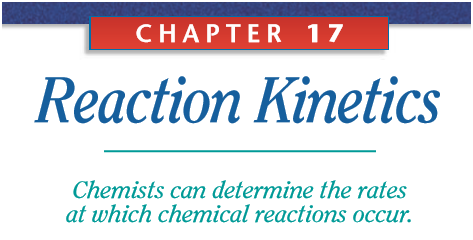# 12 GRADES SAT CHEMISTRY CH. 17 - Reaction Kinetics : Complete revision with 5 films for SAT test preparationsSection 1: The Reaction Process

Collision theory | Kinetics | Chemistry
In order for reactions to occur between substances,their particles (molecules, atoms, or ions) must collide. Furthermore, these collisions must result in interactions. The set of assumptions regarding collisions and reactions is known as collision theory. Chemists use this theory to interpret many of their observations about chemical reactions.

Collision Theory Model, Rates of Reaction, Activation Energy, Arrhenius Equation - Chemical Kinetics

This chemistry video tutorial provides a basic introduction into the collision theory model. In order for two molecules to react, they must collide with sufficient energy and with the proper molecular orientation. This video describes the relationship of the rate of the reaction with temperature and activation energy using the arrhenius equation. As the temperature increases, the rate constant k increases, which in turn increases the rate of the reaction. Whenever a catalyst is added, the activation energy decreases which increases the value of the rate constant k which in turn increases the reaction rate. This video also includes a brief discussion into energy diagrams. The forward activation energy is the difference between the energy of the transition state / activated complex and the energy of the reactants. This video is part of the chemical kinetics series. This tutorial also discusses the difference between the collision frequency, steric factor, and the frequency factor in the arrhenius equation.

Section 2: Reaction Rate

Factors Affecting the Rate of the Reaction - Chemical Kinetics

This chemistry video tutorial discusses five factors affecting the rate of a reaction. This includes the nature of the reactants, concentration, surface area, temperature, and the use of a catalyst. This tutorial is part of the chemical kinetics series

Chemical Kinetics Rate Laws – Chemistry Review – Order of Reaction & Equations

This general chemistry study guide video lecture tutorial provides an overview of chemical kinetics. It contains plenty of examples, practice problems, and conceptual questions to help you to master the course. This video is especially helpful to those taking AP chemistry in high school or general chemistry in college. Here is a list of topics: 1. How to calculate the rate of the reaction using the change in concentration and time 2. Determining the order of a reactant and the overall order of the reaction using the method of initial rates. 3. How to determine the rate equation or rate law expression 4. Calculating the rate constant K and the units of K 5. Understanding the difference between the first order, second order, and zero order reaction. 6. Equations and formulas for zero order, first, and second order reactions 7. Half Life Formula, Initial Concentration of A and Rate constant K 8. Factors affecting reaction rate – concentration, temperature, and catalyst 9. Relationship between the rate of the reaction and the concentration 10. Rate constant K, temperature, catalyst, activation energy and potential energy diagrams 11. Forward activation energy vs reverse activation energy 12. Arrhenius Equation 13. Half Life Problems and Half Life Method 14. Collision frequency, steric factor, and frequency factor 15. Reaction Mechanism – Slow Step – Rate Determining Step 16. How To Find the Intermediate and Catalyst in a Reaction Mechanism

Initial Rates Method For Determining Reaction Order, Rate Laws, & Rate Constant K, Chemical Kinetics

This chemistry video tutorial provides a basic introduction into method of initial rates which is useful for determining the order with respect to the reactants and the overall reaction order. In addition, the initial rates method is useful for experimentally determining the rate law expression for a particular chemical reaction. This video explains how to calculate the value of the rate constant K and how to determine its units. This video is part of the chemical kinetics series. It contains plenty of examples and practice problems.

ref book : HOLT

ENGINEER SAMER MAHMOUD
CHEMICAL ENGINEER
SANDS ACADEMY - AMMAN - JORDAN